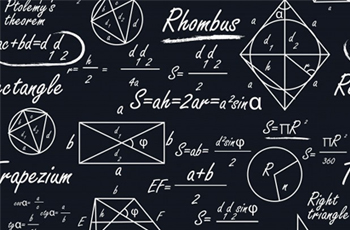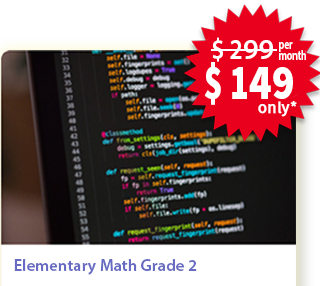## High School Geometry## Private Lessons

• 2 sessions every week
• Each session is of 1 hour
• Follow class work

#### Overview

Aligned with the common core curriculum of high school Geometry, during this course students begin to formalize their geometry experiences from elementary and middle school, using more precise definitions and developing careful proofs.

#### Curriculum

###### Congruence

• Experiment with transformations in the plane
• Understand congruence in terms of rigid motions
• Prove geometric theorems
• Make geometric constructions

###### Similarity, Right Triangles, and Trigonometry

• Understand similarity in terms of similarity transformations
• Prove theorems involving similarity
• Define trigonometric ratios and solve problems involving right triangles
• Apply trigonometry to general triangles

###### Circles

• Understand and apply theorems about circles
• Find arc lengths and areas of sectors of circles

###### Expressing Geometric Properties with Equations

• Translate between the geometric description and the equation for a conic section
• Use coordinates to prove simple geometric theorems algebraically

###### Geometric Measurement and Dimension

• Explain volume formulas and use them to solve problems
• Visualize relationships between two-dimensional and three-dimensional objects

###### Modeling with Geometry

• Apply geometric concepts in modeling situations

### Enroll for Math Private LessonsEnroll today to book your slot!

*Weekly 2 classes. Only limited seats available, Enroll Today.